Previous Page | Next Page

 Module Library

RANDNORMAL Function

generates a random sample from a multivariate normal distribution

RANDNORMAL( N, Mean, Cov )

The inputs are as follows: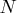is the number of desired observations sampled from the multivariate normal distribution.
Mean
is a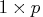vector of means.
Cov
is a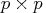symmetric positive definite variance-covariance matrix.

The RANDNORMAL function returns an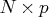matrix containingrandom draws from the multivariate normal distribution with mean vector Mean and covariance matrix Cov.

If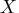follows a multivariate normal distribution with mean vector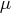and variance-covariance matrix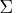, then
• the probability density function for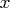is
• if
, the probability density function reduces to a univariate normal distribution.
• the expected value of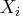is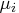.
• the covariance ofand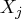is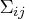.

The following example generates 1000 samples from a two-dimensional multivariate normal distribution with mean vector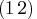, correlation matrix Corr, and variance vector Var. Each row of the returned matrix x is a row vector sampled from the multivariate normal distribution. The example then computes the sample mean and covariance and compares them with the expected values. Here are the code and the output:


call randseed(1);
N=1000;
Mean = {1 2};
Corr = {0.6 0.5,0.5 0.9};
Var = {4 9};
/*create the covariance matrix*/
Cov = Corr # sqrt(Var * Var);
x = RANDNORMAL( N, Mean, Cov );
SampleMean = x[:,];
n = nrow(x);
y = x - repeat( SampleMean, n );
SampleCov = y*y / (n-1);
print SampleMean Mean, SampleCov Cov;

SampleMean                Mean

1.0619604 2.1156084         1         2

SampleCov                 Cov

2.5513518 3.2729559         2.4       3
3.2729559 8.7099585           3     8.1


For further details about sampling from the multivariate normal distribution, see Gentle (2003, p. 197).Previous Page | Next Page | Top of Page